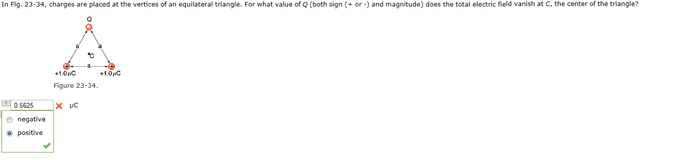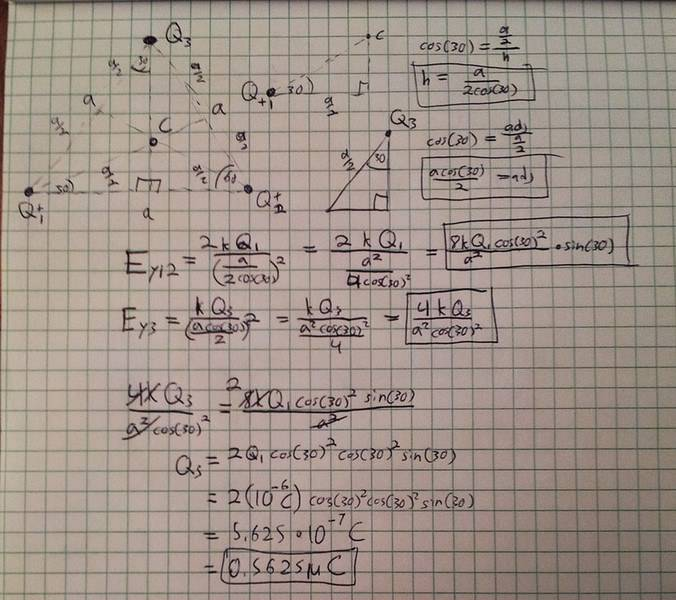# 0N/C Electric Field at center of equilateral Triangle

• Painguy

## Homework StatementI got an answer as you can see in both pictures, but apparently it is wrong. What did I do?

## The Attempt at a Solution

In this picture I found the y components of the electric field at C. Since the bottom 2 are the same i just doubled one of the electric fields. I then set it equal to the electric
field from the top to cancel out the effect from the bottom.Somehow, you are not seeing that the distance from the all the vertices to the center is the same; you do not even have to compute what it is, it will cancel out.

Note there is a much simpler solution, using only the symmetry.

The distance from vertices to the center is the same since it is an equilateral triangle. Using that information you can easily solve the problem by summing up the electrical field intensities to 0 and the distances will apparently cancel out. You don't even have to mess with trigonometrical ratios here

Last edited: